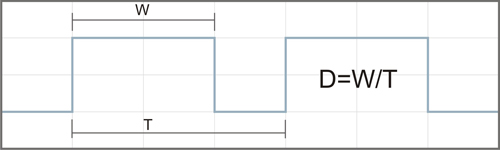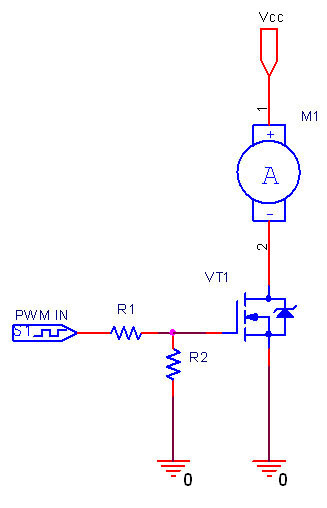# DC Motor Controller

A DC motor is an electric motor that runs on direct current (DC) electricity.

There are several possibilities to implement a DC motor controller. The rotational speed of the DC motor is proportional to the voltage applied to it. Varying the voltage you can change the motor speed.

The effective voltage (and therefore the DC motor rotational speed) can be varied by inserting a series resistor or by an electronically controlled switching device made of thyristors or transistors. All these approaches require significant changes in schematics and are hardly controlled from software.

Much better way to control the DC motor rotational speed is by using a Pulse Width Modulation (PWM). To understand how PWM can control the DC motor we should introduce the duty cycle term. The duty cycle (D) describes the proportion of positive pulse width (W) to the period (T).Current only flows through the motor during the "ON" portion of the PWM waveform, so the average voltage applied to the motor is varied by changing the duty cycle. For example, with a 100 V supply and a 30% "ON" time (D=0.3), the average voltage at the motor will be 30 V.

On the following figure you can see the PWM generator circuit for DC motor controller.DC motor controller can be implemented using the PWM functionality of DLN-Series USB-IO/I2C/SPI Interface Adapters.

Rating: